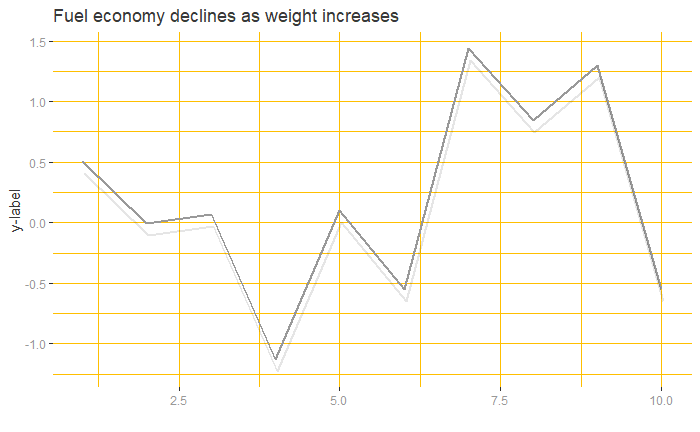# h1 Headline as an Title

### h3 Headline When you execute code within the notebook

##### h3 Headline Try executing this chunk by clicking the Run button within the chunk
df <- data.frame(x = 1:10 , y = rnorm(1:10))
ggplot(df, aes(x, y)) +
#geom_point(colour ="#999999", size = 3) +
geom_line(linetype = 1, colour ="#999999", size = 1) +
geom_line(aes(x = x+0.03, y = y-0.1), alpha = 0.1, lwd = 0.8, linetype = 1) +
theme(panel.background  = element_rect(fill = "white")) +
theme(strip.background  = element_rect(colour ="#ffbf00", fill = "#ffbf00")) +
theme(strip.text = element_text(size = rel(1.2), colour = "#393939", face = "bold")) +
theme(axis.text = element_text(colour = "#999999")) +
theme(axis.title.y = element_text(size = rel(1.0), colour = "#393939")) +
theme(axis.title.x = element_text(size = rel(1.0), colour = "#393939")) +
theme(panel.grid = element_line(colour = "#ffbf00",size = 0.1,linetype=1)) +
labs(title = "Fuel economy declines as weight increases") +
labs(y = "y-label") +
labs(x = "") +
theme(plot.title = element_text(size = rel(1.2), colour ="#393939")) #+ facet_wrap(~x)Add a new chunk by clicking the Insert Chunk button on the toolbar or by pressing Ctrl+Alt+I.

When you save the notebook, an HTML file containing the code and output will be saved alongside it (click the Preview button or press Ctrl+Shift+K to preview the HTML file).

The preview shows you a rendered HTML copy of the contents of the editor. Consequently, unlike Knit, Preview does not run any R code chunks. Instead, the output of the chunk when it was last run in the editor is displayed.